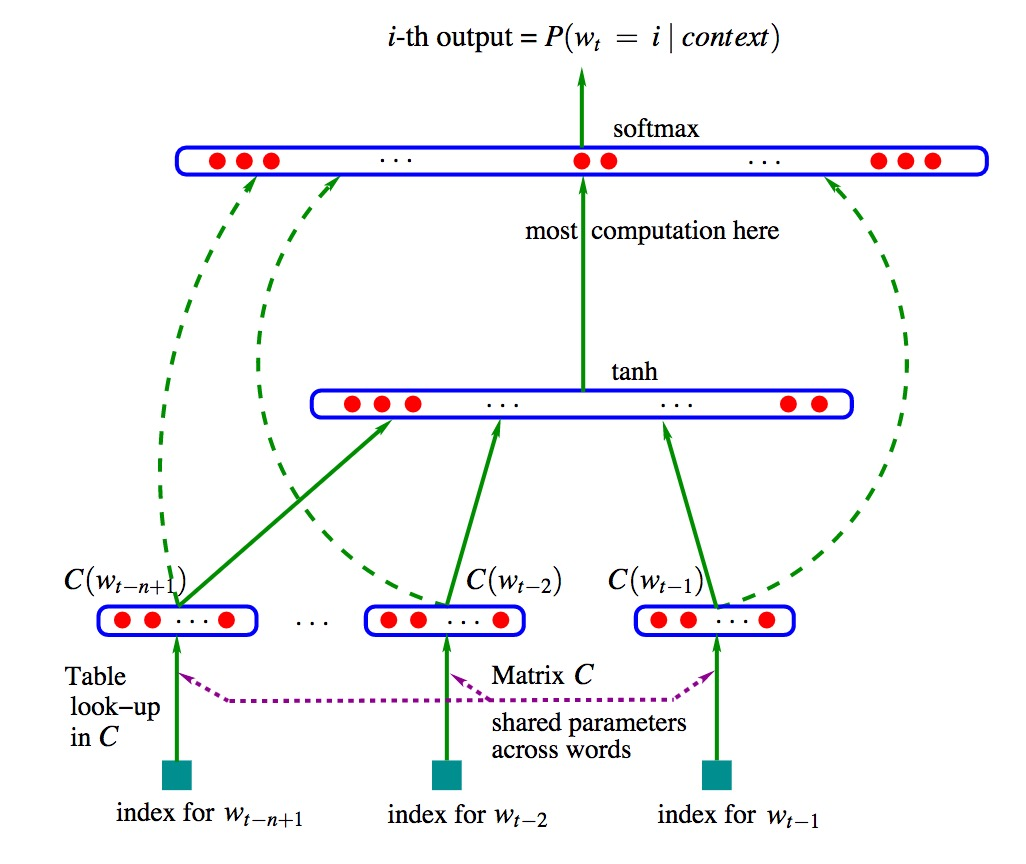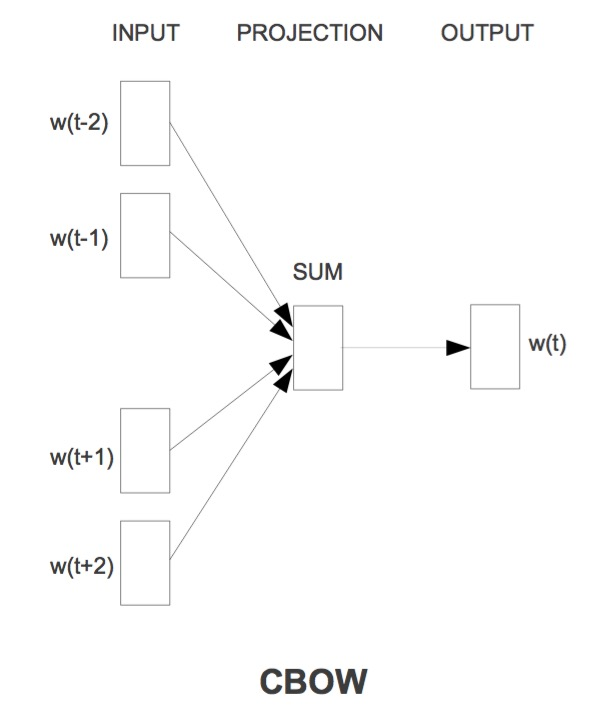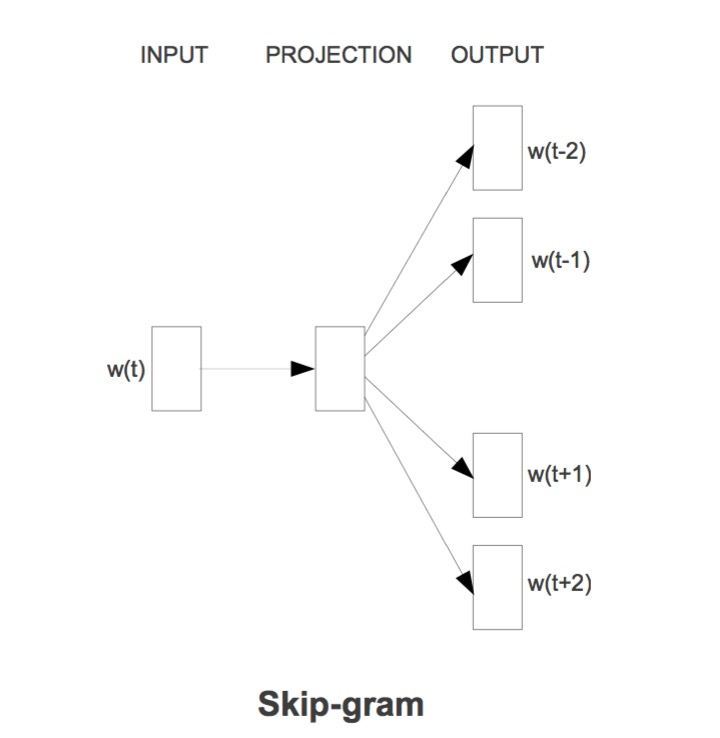# Deep Learning-word2vec

Posted by maple Blog on October 23, 2016

## 词向量

One-hot Representation

苹果 [0 0 0 1 0 0 0 0 ...]



Distributed Representation

[0.302 0.123 0.690 ...]



word2vec

## NNLM

word2vec作为一种简化的神经网络模型。在了解内部原理之前，我们需要知道下 NNLM网络结构。

NNLM 即 Neural Network Language Model，由Bengio提出《A Neural Probabilistic Language Model》$$w_{t-n+1},...w_{t-2},w_{t-1}$$表示前面n-1个词，来预测下一个词$$w_t$$.

$y=b+Wx+Utanh(d+Hx)$

$$W(V×(n-1)m)$$ 也就是上图中左边的虚线，输入层到输出层的直连。如果去掉直连，那么W参数就为0了。

$$U(V×h)$$就是隐藏层到输出层的参数

$$b(V)$$ bias项

$\theta=(b,d,W,U,H,C)$

## word2vec

### CBOW

CBOW是Continuous Bag-of-Words Model。与NNLM模型类似，不过去掉了隐藏层。$P(w_t|w_{t-k},w_{t-k+1},...w_{t+k},)$

• 在NNLM中是词向量首尾相连变成很长的一串，这里是直接求和/求平均

• 这里的求t，用了t前后的词向量，而前面是前n-1个词。似乎看起来这样更合理，因为考虑了将来的词。

• CBOW去掉了隐藏层复杂的计算。

inut到project层，来看下源代码，我加了注释。

	  //随机选择窗口大小
b = next_random % window;
if (cbow) {  //train the cbow architecture
// in -> hidden
cw = 0;
for (a = b; a < window * 2 + 1 - b; a++) if (a != window) {
//以 sentence_position 为中心 前后各 window - b 个词
c = sentence_position - window + a;
if (c < 0) continue;
if (c >= sentence_length) continue;
last_word = sen[c];
if (last_word == -1) continue;
//窗口内词向量相加
for (c = 0; c < layer1_size; c++) neu1[c] += syn0[c + last_word * layer1_size];
cw++;
}
if (cw) {
//相加的词向量取平均
for (c = 0; c < layer1_size; c++) neu1[c] /= cw;


Hierarchical Softmax

$P(w_O|w_I)=\Pi_{j=1}^{L(w)-1}\sigma([n(w,j+1)=ch(n(w,j))]*{v_{n(w,j)}^{'}}^{T} v_{wI} )$

$$[n(w,j+1)=ch(n(w,j))]$$表示$$n(w,j+1)$$是$$n(w,j)$$的一个子节点，感觉这是很显然的事，又多这么一个概念。

[x]表示x=true 则为1，否则为-1。

$$v_n$$表示Huffman树的inner(内部)节点也就是非叶子节点；

$$v_w$$表示Huffman树的叶子节点，也就是一个个词。

		//Hierarchical Softmax 也就是根据初始化的 vocab 词汇表的huffman编码来计算输出f值，
if (hs) for (d = 0; d < vocab[word].codelen; d++) {
f = 0;
l2 = vocab[word].point[d] * layer1_size;
// Propagate hidden -> output
for (c = 0; c < layer1_size; c++) f += neu1[c] * syn1[c + l2];
if (f <= -MAX_EXP) continue;
else if (f >= MAX_EXP) continue;
//f值转化到0.01～1 之间 查表expTable得到，expTable的默认大小为EXP_TABLE_SIZE＝1000
else f = expTable[(int)((f + MAX_EXP) * (EXP_TABLE_SIZE / MAX_EXP / 2))];
// 'g' is the gradient multiplied by the learning rate
// 1 - vocab[word].code[d] 表示word的第d位huffman编码 0或者1 用来表示目标值，减去输出f 计算g
g = (1 - vocab[word].code[d] - f) * alpha;
// Propagate errors output -> hidden
//g * syn1[c + l2] 梯度部分,误差回传 隐层。在后面 neu1e会继续回传到输入层，用来优化syn0
for (c = 0; c < layer1_size; c++) neu1e[c] += g * syn1[c + l2];
// Learn weights hidden -> output
for (c = 0; c < layer1_size; c++) syn1[c + l2] += g * neu1[c];



$f=\sigma(neu1^Tsyn1)$

$g = (1 - vocab[word].code[d] - f) * alpha$

1 - vocab[word].code[d] 表示word的第d位huffman编码 0或者1,比如苹果的第0位是1.

NEGATIVE SAMPLING

		//负采样 (默认采用这种方式)
//随机选择 negative 个词，如果命中 word 则 不更新误差；否则更新误差 更新网络参数
// NEGATIVE SAMPLING
if (negative > 0) for (d = 0; d < negative + 1; d++) {
if (d == 0) {
target = word;
label = 1;
} else {
next_random = next_random * (unsigned long long)25214903917 + 11;
target = table[(next_random >> 16) % table_size];
if (target == 0) target = next_random % (vocab_size - 1) + 1;
if (target == word) continue;
label = 0;
}
l2 = target * layer1_size;
f = 0;
for (c = 0; c < layer1_size; c++) f += neu1[c] * syn1neg[c + l2];
if (f > MAX_EXP) g = (label - 1) * alpha;
else if (f < -MAX_EXP) g = (label - 0) * alpha;
//查表
else g = (label - expTable[(int)((f + MAX_EXP) * (EXP_TABLE_SIZE / MAX_EXP / 2))]) * alpha;
for (c = 0; c < layer1_size; c++) neu1e[c] += g * syn1neg[c + l2];
for (c = 0; c < layer1_size; c++) syn1neg[c + l2] += g * neu1[c];


$g = (label - f) * alpha$

### Skip-gram

Skip-gram的模型图与cbow正好反一下。} else {  //train skip-gram
for (a = b; a < window * 2 + 1 - b; a++) if (a != window) {
c = sentence_position - window + a;
if (c < 0) continue;
if (c >= sentence_length) continue;
last_word = sen[c];
if (last_word == -1) continue;
l1 = last_word * layer1_size;
for (c = 0; c < layer1_size; c++) neu1e[c] = 0;
// HIERARCHICAL SOFTMAX
if (hs) for (d = 0; d < vocab[word].codelen; d++) {
f = 0;
l2 = vocab[word].point[d] * layer1_size;
// Propagate hidden -> output
// skip-gram与cbow之间的差别
// 这里的f 是直接输入 syn0 与输出syn1相乘
// 而cbow中 是输入的向量相加取平均后再作计算
// skip-gram的理念在于 用当前输入的词 word[t] 去推 上下文 word[t-2] word[t-1] word[t+1] word[t+2]的概率.
// 具体的计算目标就是优化输出syn1对应的上下文词向量
for (c = 0; c < layer1_size; c++) f += syn0[c + l1] * syn1[c + l2];
if (f <= -MAX_EXP) continue;
else if (f >= MAX_EXP) continue;
else f = expTable[(int)((f + MAX_EXP) * (EXP_TABLE_SIZE / MAX_EXP / 2))];
// 'g' is the gradient multiplied by the learning rate
g = (1 - vocab[word].code[d] - f) * alpha;
// Propagate errors output -> hidden
for (c = 0; c < layer1_size; c++) neu1e[c] += g * syn1[c + l2];
// Learn weights hidden -> output
for (c = 0; c < layer1_size; c++) syn1[c + l2] += g * syn0[c + l1];
}



1.word2vec